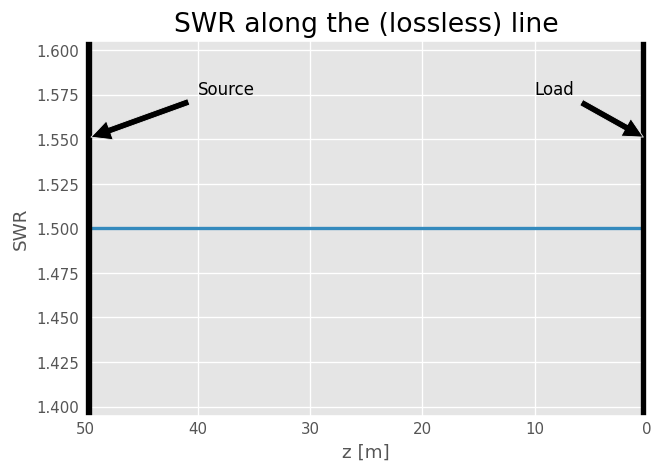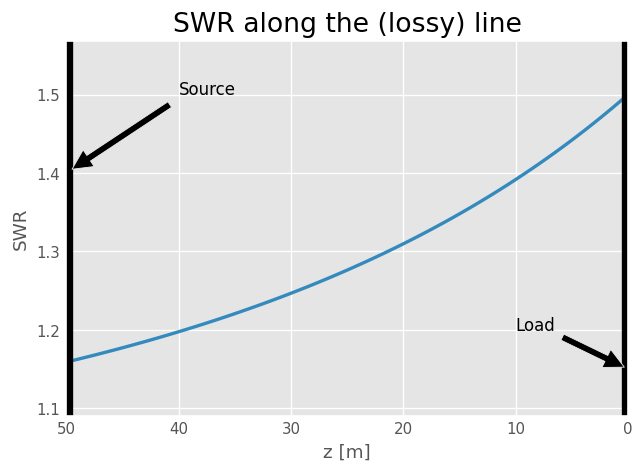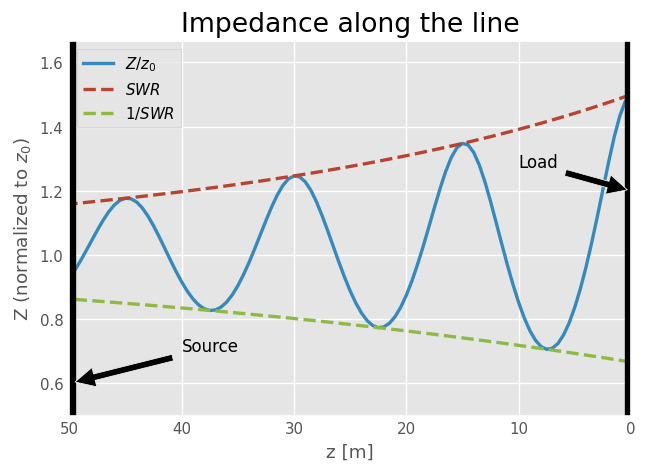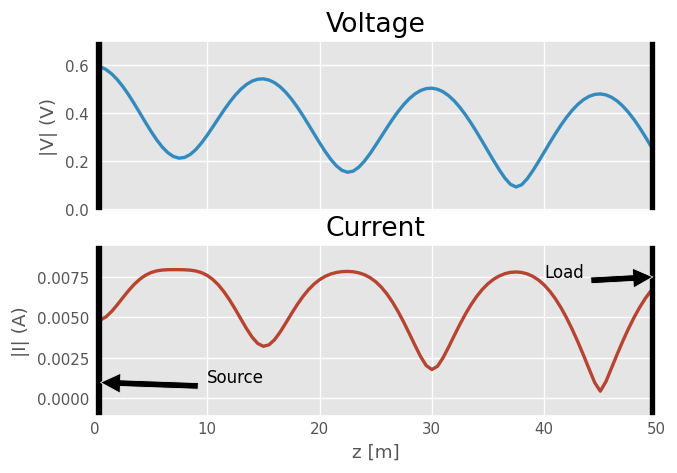# How does the SWR vary along a line?¶

Let’s assume a 10 MHz source powering an antenna (of load $$Z_L$$) through a transmission line of length $$L$$. Depending on the location of the SWR-meter, what does one would read?

This notebook is inspired from the reference: “Facts About SWR, Reflected Power, and Power Transfer on Real Transmission Lines with Loss” by Steve Stearns given at ARRL Pacificon Antenna Seminar in 2010.Let solve this question using scikit-rf. But first, the traditional Python imports:

:

%matplotlib inline

:

import matplotlib.pyplot as plt
import numpy as np
import skrf as rf

:

rf.stylely()


## Lossless lines¶

Let’s start with a lossless line of propagation constant $$\gamma=j\beta$$ and characteristic impedance $$z_0=50\Omega$$ (real).

:

freq = rf.Frequency(10, unit='MHz', npoints=1)

:

# load and line properties
Z_L = 75  # Ohm
Z_0 = 50  # Ohm
L = 50  # m

# propagation constant
beta = freq.w/rf.c
gamma = 1j*beta


Below we calculate the SWR of the line as a function of $$z$$ the line length measured from the load ($$z=0$$ at the load, $$z=L$$ at the source).

:

z = np.linspace(start=L, stop=0, num=301)
SWRs = rf.zl_2_swr(z0=Z_0, zl=rf.zl_2_zin(Z_0, Z_L, gamma*z))

:

fig, ax = plt.subplots()
ax.plot(z, SWRs, lw=2)
ax.set_xlabel('z [m]')
ax.set_ylabel('SWR')
ax.set_title('SWR along the (lossless) line')
ax.invert_xaxis()
ax.axvline(0, lw=8, color='k')
ax.axvline(L, lw=8, color='k')
arrowprops=dict(facecolor='black', shrink=0.05),
)
ax.annotate('Source', xy=(50, 1.55), xytext=(40, 1.575),
arrowprops=dict(facecolor='black', shrink=0.05),
)

:

Text(40, 1.575, 'Source')As expected, the SWR is the same everywhere along the line as the forward and backward wave amplitudes are also the same along the line.

## Lossy Lines¶

Let’s take the previous example but this time on a lossy line. The line is defined with a propagation constant $$\gamma=\alpha + j\beta$$ :

:

alpha = 0.01  # Np/m. Here a dummy value, just for the sake of the example
gamma = alpha + 1j*beta

:

z = np.linspace(0, L, num=101)
SWRs = rf.zl_2_swr(z0=Z_0, zl=rf.zl_2_zin(Z_0, Z_L, gamma*z))

:

fig, ax = plt.subplots()
ax.plot(z, SWRs, lw=2)
ax.set_xlabel('z [m]')
ax.set_ylabel('SWR')
ax.set_title('SWR along the (lossy) line')
ax.invert_xaxis()
ax.axvline(0, lw=8, color='k')
ax.axvline(L, lw=8, color='k')
arrowprops=dict(facecolor='black', shrink=0.05),
)
ax.annotate('Source', xy=(50, 1.4), xytext=(40, 1.5),
arrowprops=dict(facecolor='black', shrink=0.05),
)

:

Text(40, 1.5, 'Source')For a lossy line, the SWR is maximum at the load and decreases to be minimum at the source side.

Let’s see how the impedance varies along the line:

:

Zins = rf.zl_2_zin(Z_0, Z_L, gamma*z)

fig, ax = plt.subplots()
ax.plot(z, np.abs(Zins/Z_0), lw=2, label='$Z/z_0$')
ax.plot(z, SWRs, lw=2, ls='--', label=r'$SWR$')
ax.plot(z, 1/SWRs, lw=2, ls='--', label=r'$1/SWR$')

ax.set_xlabel('z [m]')
ax.set_ylabel('Z (normalized to $z_0$)')
ax.set_title('Impedance along the line')
ax.invert_xaxis()
ax.axvline(0, lw=8, color='k')
ax.axvline(L, lw=8, color='k')
arrowprops=dict(facecolor='black', shrink=0.05),
)
ax.annotate('Source', xy=(50, 0.6), xytext=(40, 0.7),
arrowprops=dict(facecolor='black', shrink=0.05),
)
ax.legend()

:

<matplotlib.legend.Legend at 0x7f5c1cc79fd0>The previous result is due to the fact that voltages and currents vary along the transmission line:

:

V_s = 1
Z_in = rf.zl_2_zin(Z_0, Z_L, gamma*z)
# Z_s = Z_0
V_in = V_s * Z_in/(Z_0 + Z_in)
I_in = V_in/(Z_0 + Z_in)
# note that here we are going from source to load
V, I = rf.voltage_current_propagation(V_in, I_in, Z_0, gamma*z)

:

fig, ax = plt.subplots(2,1,sharex=True)
ax.plot(z, np.abs(V), lw=2)
ax.plot(z, np.abs(I), lw=2, color='C1')
ax.set_xlabel('z [m]')
ax.set_ylabel('|V| (V)')
ax.set_ylabel('|I| (A)')
ax.set_title('Voltage')
ax.set_title('Current')
[a.axvline(0, lw=8, color='k') for a in ax]
[a.axvline(L, lw=8, color='k') for a in ax]

:

Text(10, 0.001, 'Source')